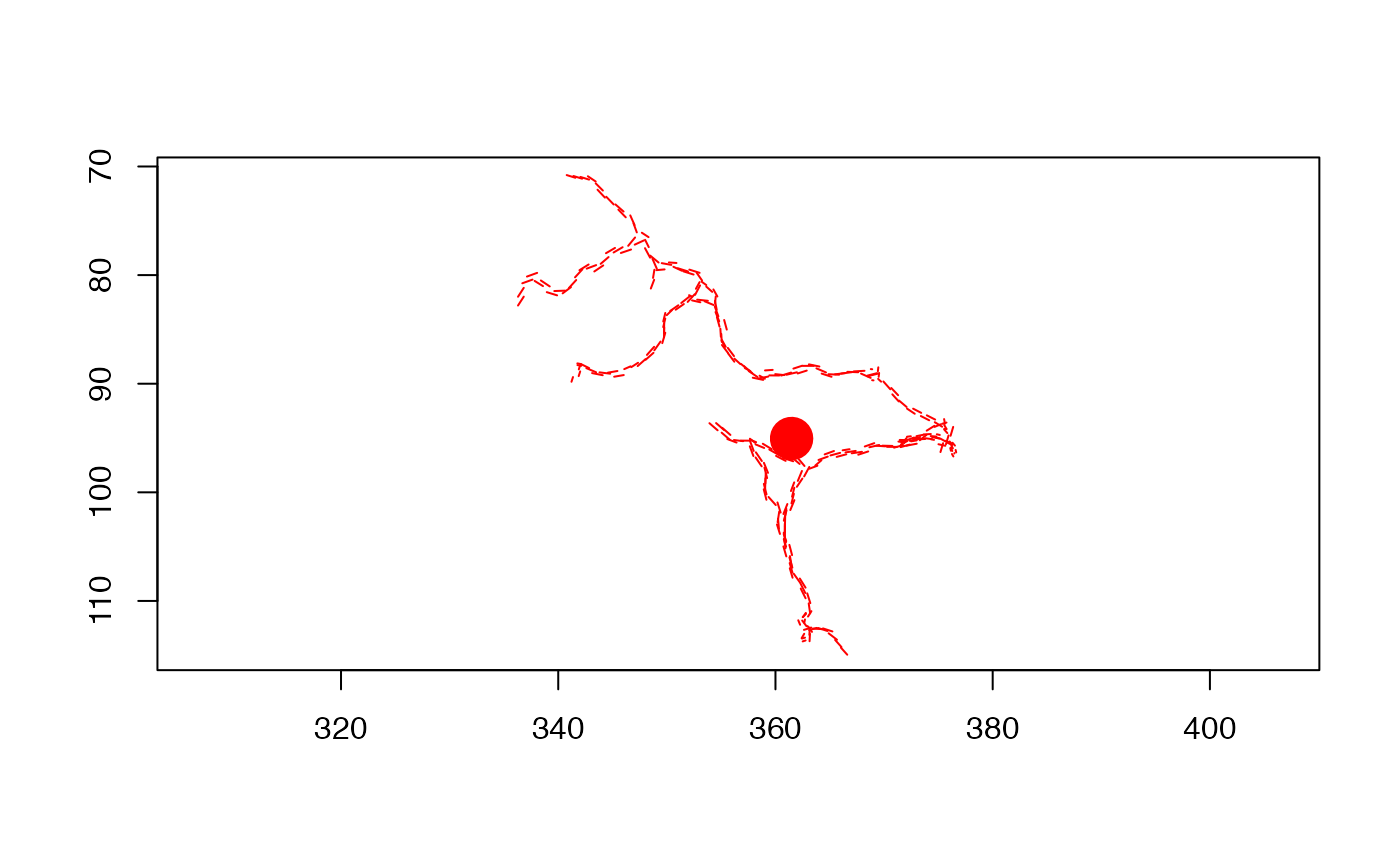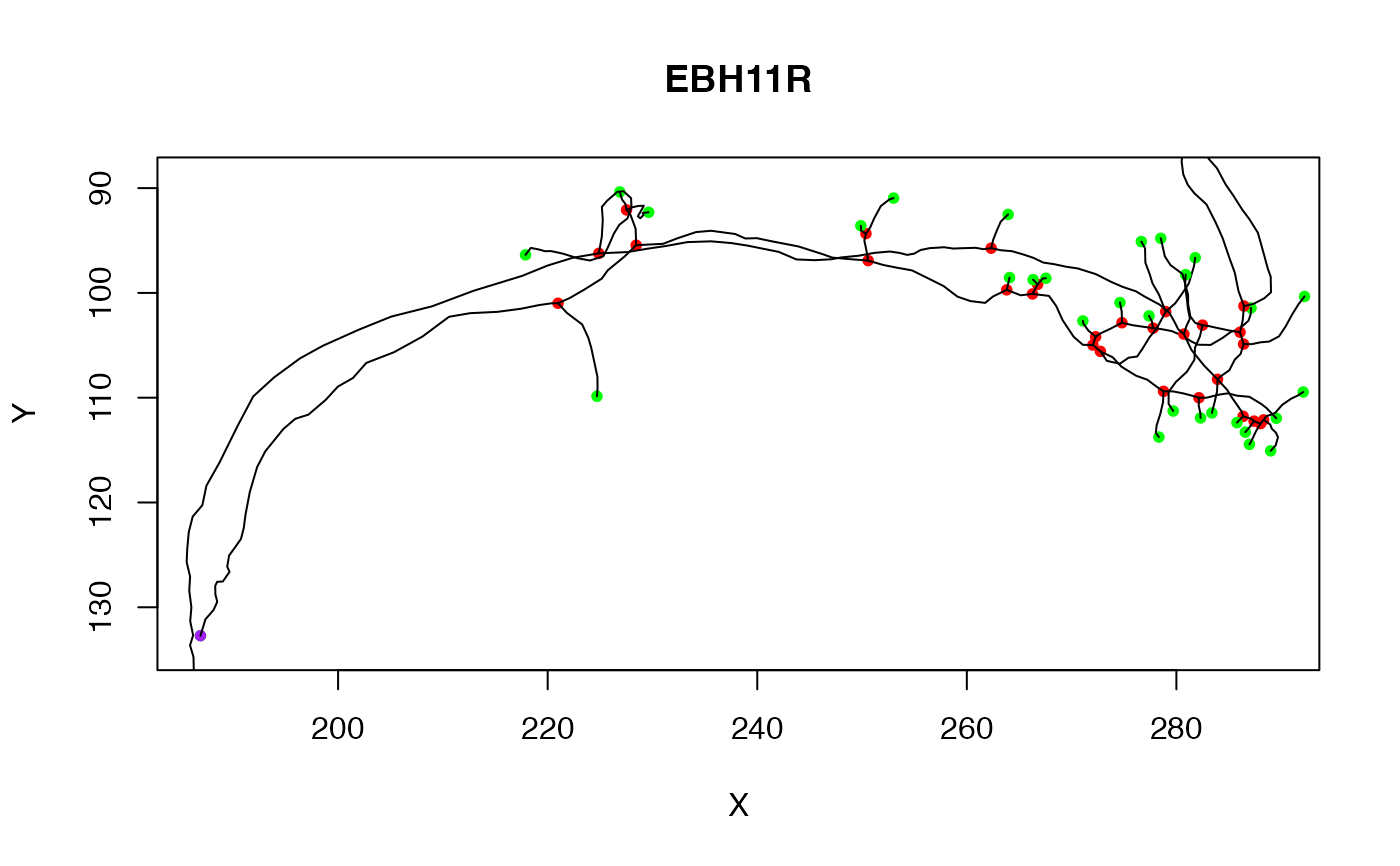plot.dotprops plots a 2D projection of a dotprops format object

plot.neuron plots a 2D projection of a neuron

# S3 method for dotprops
plot(
x,
scalevecs = 1,
alpharange = NULL,
col = "black",
PlotPoints = FALSE,
PlotVectors = TRUE,
UseAlpha = FALSE,
asp = 1,
axes = TRUE,
tck = NA,
boundingbox = NULL,
xlim = NULL,
ylim = NULL,
soma = FALSE,
...
)

# S3 method for neuron
plot(
x,
WithLine = TRUE,
WithNodes = TRUE,
WithAllPoints = FALSE,
WithText = FALSE,
PlotSubTrees = TRUE,
soma = FALSE,
PlotAxes = c("XY", "YZ", "XZ", "ZY"),
axes = TRUE,
asp = 1,
main = x\$NeuronName,
sub = NULL,
xlim = NULL,
ylim = NULL,
AxisDirections = c(1, -1, 1),
col = NULL,
PointAlpha = 1,
tck = NA,
lwd = par("lwd"),
boundingbox = NULL,
...
)

## Arguments

x

a neuron to plot.

scalevecs

Factor by which to scale unit vectors (numeric, default: 1.0)

alpharange

Restrict plotting to points with alpha values in this range to plot (default: null => all points). See dotprops for definition of alpha.

col

the color in which to draw the lines between nodes.

PlotPoints

Whether to plot points and/or tangent vectors (logical, default: tangent vectors only)

PlotVectors

Whether to plot points and/or tangent vectors (logical, default: tangent vectors only)

UseAlpha

Whether to scale tangent vector length by the value of alpha

asp

the y/x aspect ratio, see plot.window.

Whether the plot should be superimposed on one already present (default: FALSE).

axes

whether axes should be drawn.

tck

length of tick mark as fraction of plotting region (negative number is outside graph, positive number is inside, 0 suppresses ticks, 1 creates gridlines).

boundingbox

A 2 x 3 matrix (ideally of class boundingbox) that enables the plot axis limits to be set without worrying about axis selection or reversal (see details)

xlim

ylim

soma

Whether to plot a circle at neuron's origin representing the soma. Either a logical value or a numeric indicating the radius (default FALSE). When soma=TRUE the radius is hard coded to 2.

...

WithLine

whether to plot lines for all segments in neuron.

WithNodes

whether points should only be drawn for nodes (branch/end points)

WithAllPoints

whether points should be drawn for all points in neuron.

WithText

whether to label plotted points with their id.

PlotSubTrees

Whether to plot all sub trees when the neuron is not fully connected.

PlotAxes

the axes for the plot.

main

the title for the plot

sub

sub title for the plot

AxisDirections

the directions for the axes. By default, R uses the bottom-left for the origin, whilst most graphics software uses the top-left. The default value of c(1, -1, 1) makes the produced plot consistent with the latter.

PointAlpha

the value of alpha to use in plotting the nodes.

lwd

line width relative to the default (default=1).

## Value

list of plotted points (invisibly)

## Details

plot.dotprops is limited in that 1) it cannot plot somata directly (this is handled by plot.neuronlist) and 2) it can only plot a frontal (XY) view.

plot.neuron sets the axis ranges based on the chosen PlotAxes and the range of the data in x. It is still possible to use PlotAxes in combination with a boundingbox, for example to set the range of a plot of a number of objects.

nat assumes the default axis convention used in biological imaging, where the origin of the y axis is the top rather than the bottom of the plot. This is achieved by reversing the y axis of the 2D plot when the second data axis is the Y axis of the 3D data. Other settings can be achieved by modifying the AxisDirections argument.

plot3d.neuron

Other neuron: neuron(), ngraph(), potential_synapses(), prune(), resample(), rootpoints(), spine(), subset.neuron()

## Examples


plot(kcs20[], col='red')
# NB soma ignored
plot(kcs20[], col='red', soma=TRUE)
plot(kcs20, col='red', soma=TRUE)# Draw first example neuron
plot(Cell07PNs[])
# Overlay second example neuron